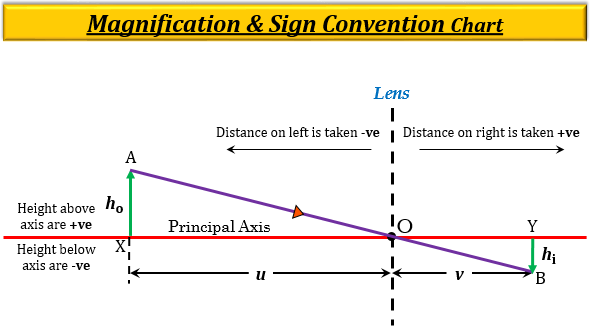# A positive sign in the value of magnification indicates that the image is ___________.

A positive sign in the value of magnification indicates that the image is virtual.

Explanation

Magnification is the increase in the image size produced by spherical mirrors concave or convex with respect to the object size.

It is considered to be the ratio of the height of an image formed by refraction from the lens to the height of an object. It is also given in terms of image distance and object which is defined as the ratio of image distance from the pole (or, optical centre) to that of object distance taken from the pole (or, optical centre). It is represented by the symbol $m$.

The magnification, $m$ produced by a spherical mirror can be expressed as:

$m=\frac{{h}_{i}}{{h}_{o}}\ or\ m=\frac{v}{u}$

So, $m=\frac{{h}_{i}}{{h}_{o}}=\frac{v}{u}$

Where,

$m$ = magnification.

${h}_{i}$ = height of image.

${h}_{o}$ = height of object.

$v$ = image distance.

$u$ = object distance.

The positive magnitude $(+)$ of magnification indicates virtual and erect image, as the virtual and erect image is formed above the principal axis which is taken as positive $(+)$ sign.

The negative magnitude $(-)$ of magnification indicates real and inverted image,  as the real and inverted image is formed below the principal axis which is taken as negative $(-)$ sign.Updated on: 10-Oct-2022

208 Views